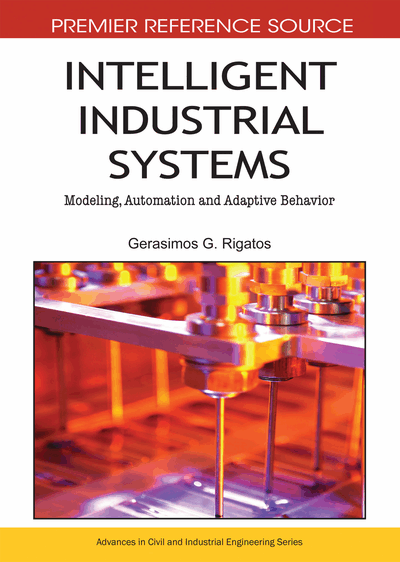# Technical Analysis and Implementation Cost Assessment of Sigma-Point Kalman Filtering and Particle Filtering in Autonomous Navigation Systems

Gerasimos G. Rigatos (Industrial Systems Institute, Greece)
DOI: 10.4018/978-1-61520-849-4.ch005
Available
\$37.50
No Current Special Offers

## Abstract

The chapter provides technical analysis and implementation cost assessment of Sigma-Point Kalman Filtering and Particle Filtering in autonomous navigation systems. As a case study, the sensor fusion-based navigation of an unmanned aerial vehicle (UAV) is examined. The UAV tracks a desirable flight trajectory by fusing measurements coming from its Inertial Measurement Unit (IMU) and measurements which are received from a satellite or ground-based positioning system (e.g. GPS or radar). The estimation of the UAV’s state vector is performed with the use of (i) Sigma-Point Kalman Filtering (SPKF), (ii) Particle Filtering (PF). Trajectory tracking is succeeded by a nonlinear controller which is derived according to flatness-based control theory and which uses the UAV’s state vector estimated through filtering. The performance of the autonomous navigation system which is based on the aforementioned state estimation methods is evaluated through simulation tests. Implementation cost assessment shows that PF requires more sample points than SPKF to approximate the state distribution. Therefore PF is a computationally more demanding method which needs more costly computing machines. However, the PF is a nonparametric filter which can be applied to any kind of state distribution, while the SPKF state estimators are still based on the assumption of a Gaussian process and measurement noise.
Chapter Preview
Top

## Introduction

The chapter provides technical analysis and implementation cost assessment of nonlinear estimation methods used in autonomous navigation systems. Since the field of terrestrial, marine and aerial autonomous navigation systems grows rapidly there is need to obtain a comparative technical analysis of the components of such systems as well as to assess their implementation cost in terms of computing power and associated hardware equipment. Nonlinear estimation based on probabilistic inference forms a core component in most modern guidance and navigation systems. The estimator fuses observations from multiple sensors with predictions from a nonlinear dynamic state-space model of the system under control. The most widely used algorithm for multi-sensor fusion is the Extended Kalman Filter (EKF), however this is based on linearization of the system dynamics which results in suboptimal application of the recursive estimation of the standard Kalman Filter (Rigatos, 2008b),(Rigatos & Tzafestas, 2007). Moreover, the EKF follows the assumption of Gaussian process/measurement noise which does not always hold. These can seriously affect the performance of the state estimation and even lead to divergence. Consequently, the performance of a control loop that uses an EKF-based estimate of the system's state vector can be unsatisfactory.

To overcome the EKF flaws, two different approaches to state estimation of nonlinear dynamical systems are proposed: (i) Sigma-Point Kalman Filters (SPKF) and particularly the Unscented Kalman Filter (UKF) (ii) Particle Filters. SPKF methods have proven to be superior to EKF in a wide range of applications. Whereas the EKF can be viewed as a first order linearization method, the SPKF achieves higher accuracy, without requiring additional computational effort. Furthermore, implementation of the SPKF is substantially easier and requires no analytic derivation or Jacobians as in the EKF case (Julier et al., 2000), (Julier & Uhlmann, 2004), (van der Merwe et al., 2004), (Sӓrkӓ, 2007), (Kandepu et al., 2008). The state distribution in SPKF is approximated by a Gaussian random variable, which is represented using a minimal set of suitably chosen weighted sample points. These sigma points are propagated through the true nonlinear system, thus generating the posterior sigma-point set, and the posterior statistics are calculated. The sample points progressively converge to the true mean and covariance of the Gaussian random variable.

The Particle Filter is a non-parametric state estimator which unlike the EKF does not make any assumption on the probability density function of the measurements (Gustafsson et al., 2005), (Arulampalam et al., 2002), (Campillo, 2006). The concept of particle filtering comes from Monte-Carlo methods. The Particle Filter (PF) has improved performance over the established nonlinear filtering approaches (e.g. the EKF), since it can provide optimal estimation in nonlinear non-Gaussian state-space models. Particle filters can approximate the system's state sufficiently when the number of particles (estimations of the state vectors which evolve in parallel) is large. The PF avoids also the calculations associated with the Jacobians which appear in the EKF equations (Rigatos, 2008a). The main stages of the PF are prediction (time update), correction (measurement update) and resampling for substituting the unsuccessful state vector estimates with those particles that have better approximated the real state vector. Comparing SPKF and PF methods, the latter require more sample points to approximate the state distribution. However, the PF is a nonparametric filter which can be applied to any kind of state distribution, while the SPKF state estimators are still based on the assumption of a Gaussian process and measurement noise.

## Complete Chapter List

Search this Book:
Reset